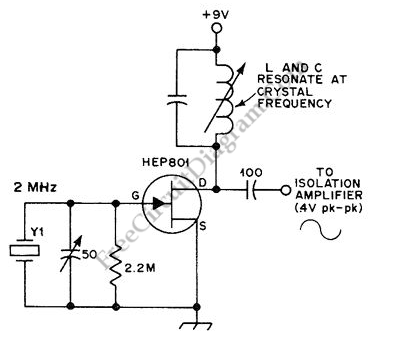## PC (Audio) Signal Generator Using RS232 Port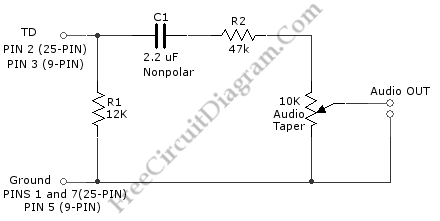Using an RS232 port, we can generate square wave oscillation when transmitting data, and this can be “misused” as signal generator (square wave generator). This is a PC Signal generator produce a square wave in audible frequency range. This circuit utilize a transmission of a “U” character from ASCII character set. This character has binary value of 01010101, making it […]

## Wien-Bridge Oscillator Using HA2541 Op-Amp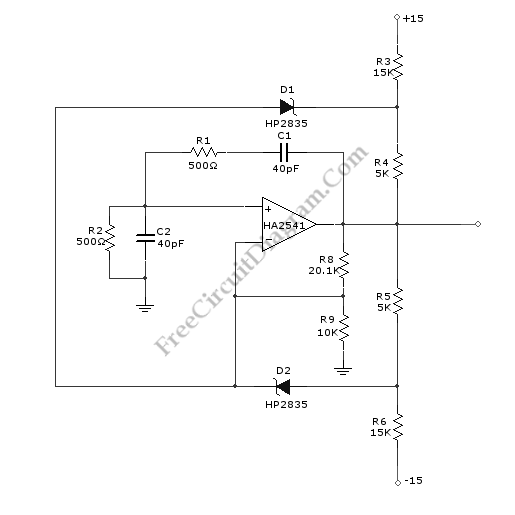A Wien-Bridge oscillator circuit can be built from HA2541 and some basic components. This circuit can generate good-quality sine wave of 40 MHz with an upper limit of 50 MHz. The R3 through R7 and D2 and D1 provide the diode limiting for this circuit. Here is the schematic diagram of this circuit: For frequency stability, this circuit need a […]

## Direct Coupled Discrete Astable Multivibrator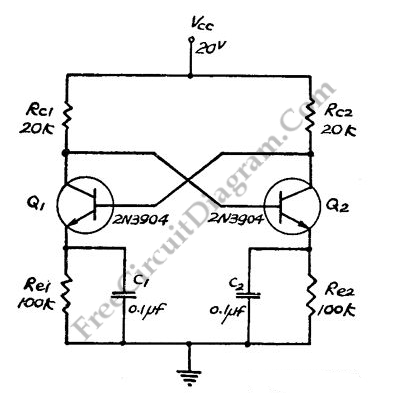This flip-flop circuit  is a free running/astable multivibrator one,  with bases and collector of both emitter biased transistor are directly coupled to each other.  Switching action is supported by means of capacitor in each emitter circuit.  This configuration produce a triangle waves at emitters. Since neither transistor can remain permanently cut off, then a free running oscillation will be generated. […]

## Variable Frequency And Duty Cycle Oscillator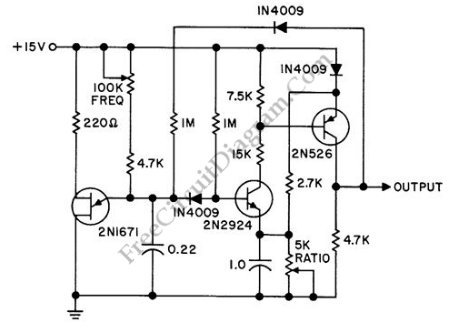A discrete oscillator circuit shown in the schematic diagram below is  a variable duty cycle and variable frequency oscillator, can be used to produce rectangular wave. This circuit has frequency range of 60 to 1,000 Hz. This circuit consist of one UJT (uni junction transistor) and two transistors that provide positive going output when emitter voltage exceeds NPN emitter voltage. […]

## Scope’s Time Base Calibrator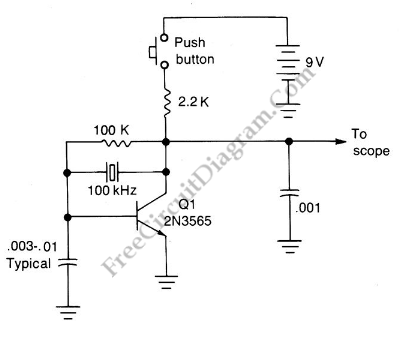A scope calibrator is a circuit that is used for calibrating the variable time base oscillator of general purpose scopes. This scope calibrator gives 100kHz reference, so if the scope is set to 10 cycles on 10 graticule divisions, then each division represents 10 microseconds or 100kHz. And each division will represent 1 Mhz or 1 microsecond if the scope […]

## 20 kHz Astable Multivibrator with Transistors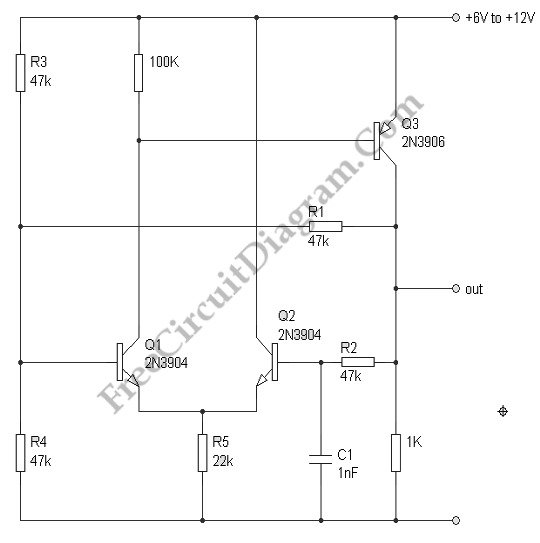Astable multivibrator or oscillator circuit is based on positive feedback. We can design such circuit using op-amp, logic gates, or transistors. Here we can rely on single capacitor capacitor and resistor for the feedback inside op-amp like transistor circuit, which works over wide range of temperature, voltages and transistor gains. The stability is pretty good, for supply voltage changes between […]